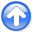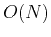First-break traveltime tomography with the double-square-root eikonal equationNext: Conclusions Up: Li et al.: DSR Previous: Synthetic Model Examples

# Discussion

There are three main issues in the DSR tomography. The first issue comes from a large dimensionality of the prestack space, which results in a considerable computational domain size after discretization. The memory consumption becomes an immediate problem for 3-D models, where the prestack traveltime belongs to a 5-D space and may require distributed storage.

The second issue is related to the computational cost. The FMM DSR we have introduced in this paper has a computational complexity of, whereis the total number of grid points after discretization,. Thefactor arises in the priority queue used in FMM for keeping track of expanding wave-fronts. Some existing works could accelerate FMM to ancomplexity and may be applicable to the DSR eikonal equation (Yatziv et al., 2006; Kim, 2001). A number of other fast methods developed for the eikonal equation might be similarly applicable to the DSR eikonal equation. These include fast sweeping (Zhao, 2005), hybrid two-scale marching-sweeping methods and various label-correcting algorithms (see Chacon and Vladimirsky (2012a) and references therein).

The last issue is possible parallelization of the proposed method. Our current implementation of the FMM DSR tomography algorithm is sequential, while the traditional tomography could be parallelized among different shots. However, we notice that the DSR eikonal equation has a plane-wave source, therefore a distributed wave-front propagating at the beginning followed by a subdomain merging is possible. A number of parllelizable algorithms for the eikonal equation have been developed (Weber et al., 2008; Detrixhe et al., 2013; Zhao, 2007; Jeong and Whitaker, 2008; Chacon and Vladimirsky, 2012b). Extending these methods to the DSR eikonal equation would be the first step in parallelizing DSR tomography.First-break traveltime tomography with the double-square-root eikonal equationNext: Conclusions Up: Li et al.: DSR Previous: Synthetic Model Examples

2013-10-16